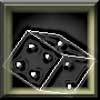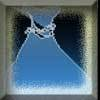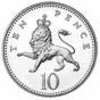#### You may also like### Gambling at Monte Carlo

A man went to Monte Carlo to try and make his fortune. Is his strategy a winning one?### Marbles and Bags

Two bags contain different numbers of red and blue marbles. A marble is removed from one of the bags. The marble is blue. What is the probability that it was removed from bag A?### Coin Tossing Games

You and I play a game involving successive throws of a fair coin. Suppose I pick HH and you pick TH. The coin is thrown repeatedly until we see either two heads in a row (I win) or a tail followed by a head (you win). What is the probability that you win?

# XOXOXO

##### Age 14 to 16 ShortChallenge Level

Using a tree diagram
The first four stages are shown in this tree diagram below, without probabilities shown. Once three of the same letter have been used, that letter can't happen again.Because the tree diagram is very large, it makes sense to consider only the branches that lead to acceptable 'words'. Those probabilities are shown below, and so are the remaining banches.

At first, there are 6 tiles to choose from, 3 of each letter. Then there are 5 tiles to choose from, and the number that there are of each letter depends on what has already been removed.So the probability of getting an acceptable 'word' is $$\left(\frac{3}{6}\times\frac{3}{5}\times\frac{2}{4}\times\frac{2}{3}\times\frac{1}{2}\times\frac{1}{1}\right)+\left(\frac{3}{6}\times\frac{3}{5}\times\frac{2}{4}\times\frac{2}{3}\times\frac{1}{2}\times\frac{1}{1}\right)\\ =\frac{1}{2}\times\frac{3}{5}\times\frac{1}{2}\times\frac{2}{3}\times\frac{1}{2}\times2\\ =\frac{3\times2\times2}{2\times5\times2\times3\times2}\\ =\frac{1}{2\times5}=\frac{1}{10}$$

When the second tile is placed, there are 5 tiles to choose from, and 3 of them are different to the first tile, because they are the letter that has not been used yet. So the probability that the first two letters are acceptable is $\frac{3}{5}$.
When the third tile is placed, there are 4 tiles to choose from - 2 of each letter. The third tile must not be the same as the second tile, so the probability that the third letter is acceptable is $\frac{2}{4}=\frac{1}{2}$.
When the fourth tile is placed, there are 3 tiles to choose from, and 2 of them are different to the third tile, because they are the letter that was used as the third tile. So the probability that the fourth letter is acceptable is $\frac{2}{3}$.
When the fifth tile is placed, there are only 2 tiles to choose from. The fifth tile must not be the same as the fourth tile, so the probability that the fifth letter is acceptable is $\frac{1}{2}$. Then there is only 1 tile left, and it will be the other letter, which is acceptable.
So the probability of getting an acceptable 'word' is $\frac{3}{5}\times\frac{1}{2}\times\frac{2}{3}\times\frac{1}{2}=\frac{1}{10}$.